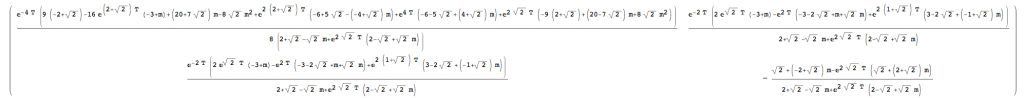ECE 515, Homework 5

$$\def\Res{\mathtt{Res}}\def\Real{\mathbb{R}}$$

1. Consider optimal control problem for $$x\in\Real, u\in\Real$$:
$\dot{x}=u,\quad J_0^T=\int_0^T\frac{u^2}{2}dt +\cos(x(T))\to\min.$

• 
Find maximal $$T$$ for which the cost function (referred to as $$S$$ in the course notes) at time $$0$$,
$V(x, 0)=inf_{\{u(t)\}_{0\leq t\leq T}, x(0)=x}\ J_0^T$
is smooth as a function of the initial point $$x$$ (i.e. has continuous derivative).

Solution. As we discussed, the cost function for the problem above is given by
$V(x,0)=\min_X C(x,X,T), \mathrm{where} C(x,X,T)=\frac{(x-X)^2}{2T}+\cos(X)$
(here $$X=x(T)$$ is the potential position of the end of the trajectory, and $$(x-X)^2/2T$$ is the minimal cost of reaching it from $$x$$). The cost function loses smoothness when $$C$$ acquires non-unique minimum for some $$x$$. This can happen only if $$C$$ becomes nonconvex (in $$X$$, i.e. when the second derivative
$\frac{d^2C}{dX^2}=\frac1T-\cos(X)$
vanishes somewhere. This happens at $$T=1$$.

• 
Describe $$V(0,0)$$ as a function of $$T$$.

Solution. We are looking at
$V(0,0)=\min_X \frac{(X)^2}{2T}+\cos(X)$
For $$T\leq 1$$ this is a convex function (of $$X$$), having vanishing derivative at $$0$$, and the corresponding minimum attained at $$0$$ is $$1$$.
For $$T\gt 1$$, there are two competing (global) minima, at point $$X(T)$$ solving
$X(T)/T=\sin(X(T)), 0\lt X(T)\lt \pi$
(there is no closed form expression for it), and the resulting cost, as a function of $$T$$, is shown below. Note that it converges to $$-1$$ as $$T\to\infty$$.

2. Consider LQR problem with
$\dot{x}=Ax+bu, J=\int_0^T |c x|^2+r|u|^2 dt +m|x(T)|^2,$
where
$A=\left( \begin{array}{cc}-2&0\\0&1\end{array} \right), b=\left(\begin{array}{c}0\\1\end{array}\right),c=(1\quad 1).$

1.  Solve corresponding Riccati differential equation for $$T=1, r=1, m=0$$;
2. 
Same for $$T=1, r=1, m=-6$$. Is the solution regular (bounded, smooth) for all $$t$$?
3. 
Find smallest $$m$$ for which the RDE with $$P(T)=mE$$ – with $$E$$ the identity matrix, – is regular for any $$T$$.

Solution:
One constructs, in the usual fashion, the Hamiltonian matrix
$H=\left( \begin{array}{cccc} -2&0&0&0\\0&1&0&-1\\-1&-1& 2& 0\\-1& -1& 0& -1 \end{array} \right)$

Using computer algebra, one can find $$\exp(-T H)$$, and apply it to the $$2n\times n$$ matrix $$E;mE$$, corresponding to the terminal value of $$X,Y$$. Then one can find $$P=YX^{-1}$$ – the solution of RDE at $$t=0$$. The result is somewhat long:$$P$$ matrix at $$t=0$$. For general $$t$$, replace $$T$$ by $$T-t$$.

To check, whether \P\) is bounded, it is enough to check that $$\det X$$ does not vanish.

Again, using computer algebra we get
$\det X(t)=e^{(2 – \sqrt{2}) T} (2 + \sqrt{2} – \sqrt{2} m + e^{2 \sqrt{2} T} (2 – \sqrt{2} + \sqrt{2} m))/4.$

We see that for $$m=0$$, the determinant is positive for all $$T$$, while for $$m=-6$$ it is vanishing at
$T-t=\frac{1}{2\sqrt{2}}\log{\frac{2+7\sqrt{2}}{7\sqrt{2}-2}}\approx .14485…$

It is immediate that $$\det X$$ vanishes for some $$T-t>0$$ iff
$m=-\frac{\sqrt{2}+1-e^{2 \sqrt{2}(T-t)}(\sqrt{2}-1)}{e^{2 \sqrt{2}(T-t)}-1}.$
As $$e^{2 \sqrt{2}(T-t)}$$ varies from $$1$$ to $$\infty$$, the values of $$m$$ that could lead to vanishing of $$\det X$$ lie in the interval $$(-\infty,-\sqrt{2}+1)$$. Thus the smallest $$m$$ ensuring regularity of $$P$$ is $$-\sqrt{2}+1$$.

15 Responses to ECE 515, Homework 5

1. Nicholas Alan Thompson December 5, 2018 at 8:43 am #

In the Problem 2 cost function, should |c*x| be |cx| so the dimensions match?

• yuliy December 5, 2018 at 1:03 pm #

It should, and it is, – belatedly, – now. Thanks!

2. Austin Hamlett December 7, 2018 at 10:26 am #

How would we solve |x(T)|^2 if x is a 2×1 column vector?

• Nicholas Alan Thompson December 7, 2018 at 10:59 am #

Think of |x(T)| as the norm, rather than the absolute value.

3. Anthony S Podkowa December 8, 2018 at 2:43 pm #

How are you defining “regular” in this context? I didn’t see anything in Basar’s notes, but it’s possible I missed something.

• yuliy December 8, 2018 at 3:28 pm #

Not exploding…

4. Anthony S Podkowa December 8, 2018 at 2:54 pm #

Can you be more explicit about the arguments of V in problem 1? The notes use a number of different conventions, with variations on x, u, and t.

• yuliy December 8, 2018 at 3:28 pm #

Updated.

• Heng-Sheng Chang December 9, 2018 at 11:17 am #

I thought $$V(x,0)$$ is minimizing $$J_0^T$$ with respect to $$u(t)$$ and $$x(T)$$, and therefore, $$V(x,0)$$ will be a function of $$x(0)$$. But the definition of $$V(x,0)$$ stated in problem 1.a is minimizing $J_0^T$ with respect to $$u(t)$$ and $$x(0)$$, then $$V(x,0)$$ will not be a function of $$x(0)$$. Not sure at which part i am getting it wrong.

• Heng-Sheng Chang December 9, 2018 at 11:22 am #

I thought $$V(x,0)$$ is minimizing $$J_0^T$$ with respect to $$u(t)$$ and $$x(T)$$, and therefore, $$V(x,0)$$ will be a function of $$x(0)$$. But the definition of $$V(x,0)$$ stated in problem 1.a is minimizing $$J_0^T$$ with respect to $$u(t)$$ and $$x(0)$$, then $$V(x,0)$$ will not be a function of $$x(0)$$. Not sure at which part I am getting it wrong.

5. Tixian Wang December 8, 2018 at 8:43 pm #

Isn’t the problem 2 too complicated to do hand calculation? It’s frustrating…… Using matlab doesn’t work either because the result is to complicated in variables. Is there a simpler way to deal with p2?

6. yuliy December 8, 2018 at 9:47 pm #

The result is moderately complicated, but does involve normal form of a 4×4 matrix, so that I expect some computer assistance.

• Tixian Wang December 8, 2018 at 10:19 pm #

Thanks

7. Tixian Wang December 8, 2018 at 10:16 pm #

For Problem 1 (b), is there an analytical solution to V(0,0) or do we just need to plot it?

• yuliy December 9, 2018 at 12:23 pm #

No close form solution is known; just describe the minimizers and sketch the value of the function.